# Test: Intercept Form And Family Of Planes

## 5 Questions MCQ Test Mathematics (Maths) Class 12 | Test: Intercept Form And Family Of Planes

Description
This mock test of Test: Intercept Form And Family Of Planes for JEE helps you for every JEE entrance exam. This contains 5 Multiple Choice Questions for JEE Test: Intercept Form And Family Of Planes (mcq) to study with solutions a complete question bank. The solved questions answers in this Test: Intercept Form And Family Of Planes quiz give you a good mix of easy questions and tough questions. JEE students definitely take this Test: Intercept Form And Family Of Planes exercise for a better result in the exam. You can find other Test: Intercept Form And Family Of Planes extra questions, long questions & short questions for JEE on EduRev as well by searching above.
QUESTION: 1

### The equation of plane through the intersection of planes (x+y+z =1) and (2x +3y – z+4) =0 is​

Solution:

The equation of any plane passing through the line of intersection of two planes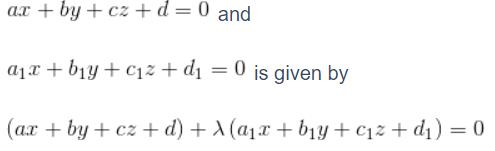As we have learnt from the concept Equation of plane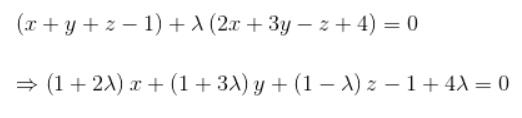QUESTION: 2

### The equation of the plane passing through the line of intersection of the planes x-2y+3z+8=0 and 2x-7y+4z-3=0 and the point (3, 1, -2) is:​

Solution:

(x - 2y + 3z + 8) + μ(2x - 7y + 4z - 3) = 0
i.e, (1 + 2μ)x - (2 + 7μ)y + (3 + 4μ)z + (8 - 3μ) = 0......(1)
the required plane is passing through (3, 1, -2)
so, 3(1 + 2μ) - (1)(2 + 7μ) + (-2)(3 + 4μ)+ (8 - 3μ) = 0
3 + 6μ - 2 - 7μ -6 -8μ + 8 - 3μ = 0
by solving, μ = 1/4
putting μ in equation (1)
we get the required equation of plane as :- 6x - 15y + 16z + 29 = 0

QUESTION: 3

### If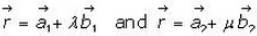are the equations of two given lines the, what is the condition for coplanarity of the twi lines in the vector form.

Solution:
QUESTION: 4

The equation of the plane which makes the intercepts 3, 4, 12 with X-axis, Y-axis and Z-axis respectively is:​

Solution:
QUESTION: 5

The equation of the plane passing through the intersection of the planes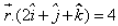and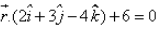and the point (1, 2, 1) is:​

Solution:

n1 = 2i + j + k
n2 = 2i + 3j - 4k
p1 = 4,   p2 = -6
r.(n1 + λn2) = p1 + λp2
=> r . [2i + j + k + λ(2i + 3j - 4k)] = 4 - 6λ
=> r . [ i(2 + 2λ) + j(1 + 3λ) + k(1 - 4k)] = 4 - 6λ
Taking r = xi + yj + zk
(2 + 2λ)x + (1 + 3λ)y + (1 - 4k)z = 4 - 6λ
(2x + y + - z - 4) + λ(2x + 3y - 4k + 6) = 0
Given points are (1,2,1)
(2 + 2 - 1 - 4) + λ(2 + 6 - 4 + 6) = 0
-1 + λ(10) = 0
λ = 1/10
Substitute  λ = 1/10, we get
18x + 7y + 14z - 46=0